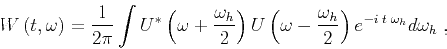Interferometric imaging condition for wave-equation migration## Wigner distribution functions

Consider the complex signalwhich depends on time. By definition, its Wigner distribution function (WDF) is (Wigner, 1932):(26)

wheredenotes temporal frequency,denotes the relative time shift of the considered signal relative to a reference timeand the signdenotes complex conjugation of complex signal. The same WDF can be obtained in terms of the spectrumof the signal:(27)

wheredenotes the relative frequency shift of the considered spectrum relative to a reference frequency. The time integral in equation C-1 or the frequency integral in equation C-2 spans the entire domain of time and frequency, respectively. When the interval is limited to a region around the reference value, the transformation is known as pseudo Wigner distribution function.

A special subset of the transformation equation C-1 corresponds to zero temporal frequency. For input signal, we obtain the output Wigner distribution functionas(28)

The WDF transformation can be generalized to multi-dimensional signals of space and time. For example, for 2D real signals function of space,, the zero-wavenumber pseudo WDF can be formulated as(29)

whereanddenote relative shift of the signalrelative to positionsand, respectively. In this particular form, the pseudo WDF transformation has the property that it filters the input of random fluctuations preserving in the output image the spatially coherent components in a noise-free background.

For illustration, consider the model depicted in Figure 1(a). This model consists of a smoothly-varying background withrandom fluctuations. The acoustic seismic wavefield corresponding to a source located in the middle of the model is depicted in Figure 1(b). This wavefield snapshot can be considered as the random image''. The application of the 2D pseudo WDF transformation to images shown in Figure 1(b) produces the image shown in Figure 1(c). We can make three observations on this image: first, the random noise is strongly attenuated; second, the output wavelet is different from the input wavelet, as a result of the bi-linear nature of the pseudo WDF transformations; third, the transformation is isotropic, i.e. it operates identically in all directions. The pseudo WDF applied to this image usesgrid points in the vertical and horizontal directions. As indicated in the body of the paper, we do not discuss here the optimal selection of the WDF window. Further details of Wigner distribution functions and related transformations are discussed by Cohen (1995).ss,wfl-6,wdf-6
Figure 12.
Random velocity model (a), wavefield snapshot simulated in this model by acoustic finite-differences (b), and its 2D pseudo Wigner distribution function (c).Interferometric imaging condition for wave-equation migration